This notebook contains code and some discussion created to address the stackexchange question

## Can a car get better mileage driving over hills?¶

Two towns are at the same elevation and are connected by two roads of the same length. One road is flat, the other road goes up and down some hills. Will an automobile always get the best mileage driving between the two towns on the flat road versus the hilly one, if operated by a perfect driver with knowledge of the route? Is there any hilly road on which a better mileage can be achieved?

In :
import numpy as np
import scipy as sp
import matplotlib.pyplot as plt
%matplotlib inline


# Car Model¶

We begin by defining some parameters for our car model, from an EPA report for a 2004 Honda Civic.

In :
A = 105.47  #N
B = 5.4276 #N/mps
C = 0.267 #N/mps^2
m = 1239 #kg
g = 9.8 #m/s^2
a = 3.19 #0.15 * g
b = 7.193 #0.6 * g
P0 = 6000 # Watts

mphfact = 2.237
gallonperj = 9.478e-4 / 114000.0 / 0.34


This hypothetical vehicle feels a friction force, a rotating friction, and air drag

$$f = A (v > 0) + B v + C v^2$$

at constant velocity, the power consumed by the vehicle will be $$P = P_0 + Fv = P_0 + A v + B v^2 + C v^3$$ if we are concerned with the energy to go some constant distance, we have that $$U = \int dt\, P = \int dx\, \frac{dt}{dx} P = \Delta x \frac{P}{v}$$ so that our fuel efficiency is given by $$\frac{\Delta x}{U} = \frac{v}{ P }$$

using the numbers from the EPA report, we can see the fuel efficiency of this vehicle

In :
vs = np.linspace(1, 90/mphfact, 300)
mpg = vs/( A*vs + B*vs**2 + C*vs**3 + P0 ) / 1609 / gallonperj
plt.plot( vs * mphfact, mpg, lw=3, alpha=0.8 );
plt.xlabel("v (mph)");
plt.ylabel("mpg");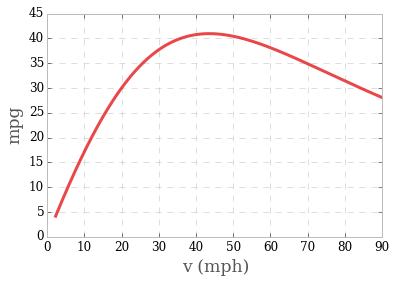I've actually cheated a bit, and picked $P_0$ to give a maximum fuel efficiency near 50 mph. In either case, we now have a decent model of a car's fuel consumption.

Or in the more useful gallons/100 miles

In :
plt.plot( vs * mphfact, 100./mpg, lw=3, alpha=0.8 );
plt.xlabel("v (mph)");
plt.ylabel("gallons/(100 miles)");
plt.ylim((0,5));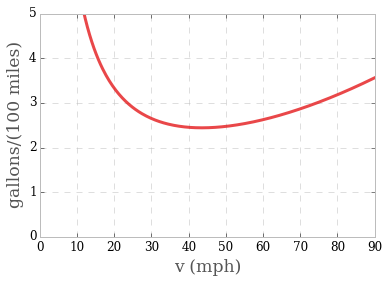The peak efficiency of our model car is:

In :
from scipy.optimize import minimize
minimize( lambda v: -v/( A*v + B*v**2 + C*v**3 + P0 ), 25 ).x * mphfact

Out:
41.421056713604862

# Dynamic Programming for Flat Terrain¶

We need to define the acceleration and fuel consumption of our car

In :
# the acceleration of our car
def f(v,u):
return u - A/m*(v>0) - B/m*v - C/m*v**2

#the power consumption of the car
def r(v,u):
return m*v*u*(u>0) + P0


Now we use dynamic programming to find an optimal control trajectory for our vehicle, on a grid of positions and possible velocities.

In :
# define our grid
XNUM = 161
VNUM = 100
VMAX = 50/mphfact
dx = 10

# create our dynamic programming variables
costs = np.zeros((XNUM, VNUM))
uvals = np.zeros((XNUM, VNUM))
args = np.zeros((XNUM, VNUM))
vs = np.linspace(0, VMAX, VNUM)

# set the end conditions
costs[-1,:] = np.inf
costs[-1,0] = 0

# fill in all of the other values
for i in xrange(costs.shape-2,-1,-1):
# for each position going backwards
for j,vim1 in enumerate(vs):
# for each of our discritized velocities, compute the optimal cost
v = 0.5*(vs + vim1)  #candidate average velocities
dv = vs - vim1  # candidate differences in velocities
u = ( v * dv + 0.5 * (dv)**2 )/dx + A/m * (v>0) + B/m * v + C/m * v**2  # candidate values of u
u[np.isnan(u)] = 0  # fix broken values at 0
dcost = r(v, u)/v  # compute all of the candidate costs
# apply our physical constraints on u
constraint = np.piecewise( u, [ u < -b, (u <= a)*(u >= -b), u > a ], [ np.inf, 0, np.inf ])
# all possible new costs
newcost = costs[i+1,:] + dcost*dx + constraint
# take the best one
costs[i,j] = newcost.min()
uvals[i,j] = u[newcost.argmin()]
args[i,j] = newcost.argmin()

/home/alemi/anaconda/lib/python2.7/site-packages/IPython/kernel/__main__.py:26: RuntimeWarning: divide by zero encountered in divide


Having computed all of the possible costs for all possible $x$s and $v$s,

In :
xgrid,vgrid = np.mgrid[0:dx*XNUM:XNUM*1j,0:VMAX:VNUM*1j]
galloncosts = costs*gallonperj
plt.pcolor( xgrid/1609.0, vgrid*mphfact, galloncosts, vmin=0, vmax=galloncosts[np.isfinite(costs)].max(), cmap='cubehelix' );
plt.xlim((0,dx*XNUM/1609));
plt.ylim((0,VMAX*mphfact));
plt.ylabel("v (mph)");
plt.xlabel("x (miles)");
plt.title("Fuel cost for optimal path (gallons)");
plt.colorbar();
plt.tight_layout();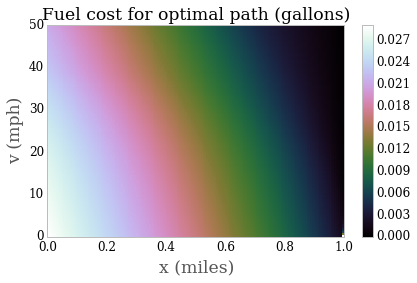Now we want to extract the path we are interested in, namely $C(0,0)$. For this we need to trace through all of the optimal arguments at each step

In :
traceargs = np.zeros(XNUM)
traceargs = 0
for i in xrange(1,XNUM):
traceargs[i] = args[i-1,traceargs[i-1]]

traceu = np.array([ uvals[i,arg] for i,arg in enumerate(traceargs) ])
tracev = np.array([ vs[arg] for i,arg in enumerate(traceargs) ])
tracecost = np.array([ costs[i,arg] for i,arg in enumerate(traceargs) ])

In :
v = 0.5*(tracev[:-1] + tracev[1:])
dv = tracev[1:] - tracev[:-1]
vdot = f(v, traceu[:-1])
dt = dx / v
ts = dt.cumsum()
print ts.max()
xs = np.linspace(0, XNUM*dx, XNUM)

108.905442186

In :
# Convience routines for a nice plot
# FROM: http://stackoverflow.com/questions/10481990/matplotlib-axis-with-two-scales-shared-origin
"""shift axis ax by ydiff, maintaining point v at the same location"""
inv = ax.transData.inverted()
_, dy = inv.transform((0, 0)) - inv.transform((0, ydif))
miny, maxy = ax.get_ylim()
miny, maxy = miny - v, maxy - v
if -miny>maxy or (-miny==maxy and dy > 0):
nminy = miny
nmaxy = miny*(maxy+dy)/(miny+dy)
else:
nmaxy = maxy
nminy = maxy*(miny+dy)/(maxy+dy)
ax.set_ylim(nminy+v, nmaxy+v)

def align_yaxis(ax1, v1, ax2, v2):
"""adjust ax2 ylimit so that v2 in ax2 is aligned to v1 in ax1"""
_, y1 = ax1.transData.transform((0, v1))
_, y2 = ax2.transData.transform((0, v2))

In :
# plot v(x), u(x)
fig,ax = plt.subplots()
c1 = "#e41a1c"
c2 = "#377eb8"
ax.plot( xs, tracev*mphfact, lw=2, alpha=0.8, c=c1 );
ax.set_xlabel(r"$x$ (mph)");
for tl in ax.get_yticklabels():
tl.set_color(c1)
ax.set_ylabel(r"$v(x)$ (m/s)", color=c1);
ax2 = ax.twinx()
ax2.plot( xs, traceu, lw=2, alpha=0.8, c=c2 );
for tl in ax2.get_yticklabels():
tl.set_color(c2)
ax2.set_ylabel(r"$u(x)$ (m/s$^2$)", color=c2);
ax.set_xlim((0,1609));
ax2.grid('off');
align_yaxis(ax,0,ax2,0);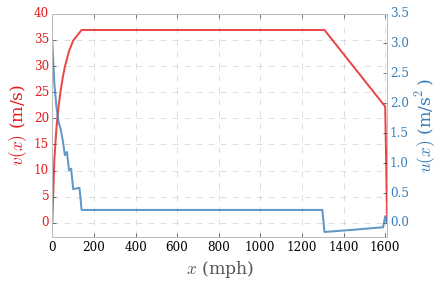In :
# plot v(t) and x(t)
fig,ax = plt.subplots()
c1 = "#e41a1c"
c3 = "#984ea3"
tts = np.r_[0,ts]
ax.plot( tts, tracev*mphfact, lw=2, alpha=0.8, c=c1 );
ax.set_xlabel(r"$t$ (s)");
for tl in ax.get_yticklabels():
tl.set_color(c1)
ax.set_ylabel(r"$v(t)$ (m)", color=c1);
ax2 = ax.twinx()
ax2.plot( tts, xs, lw=2, alpha=0.8, c=c3 );
for tl in ax2.get_yticklabels():
tl.set_color(c3)
ax2.set_ylabel(r"$x(t)$ (m/s)", color=c3);
ax.set_xlim((0,ts.max()));
ax2.grid('off');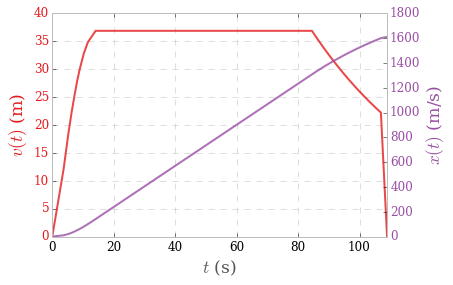In :
# plot fuel consumption
fig,ax = plt.subplots()
c4 = "#4daf4a"
consump = r(v, traceu[:-1])/v * dx * 100 * gallonperj
ax.plot( xs[:-1]/1609, consump, lw=3, alpha=0.8, c=c4 );
ax.set_ylabel("fuel use (gallons/100 mi)");
ax.set_xlabel("x (mi)");
gallons = consump.sum()/100.0
mpg = 100./consump.sum()
ax.set_title("tot={:0.2g} ga={:.1f} mpg".format(gallons, mpg));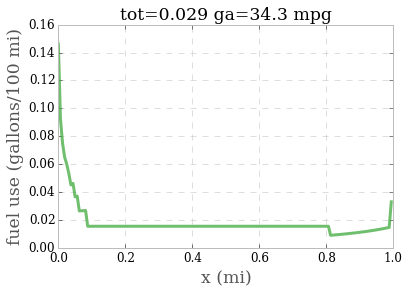In :
#plot -a/g
fig,ax = plt.subplots();
c5="#a65628"
ax.plot( xs/1609, np.r_[0,(-f(v,traceu[:-1])*dx/g).cumsum()], lw=3, alpha=0.8, c=c5 );
ax.set_xlim((0,1));
ax.set_xlabel("x (mi)");
ax.set_ylabel(r"$\int\, dx\, \left(-\frac{a}{g}\right)$  (m)");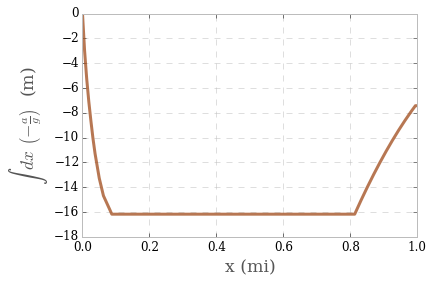## Dynamic Program for Arbitrary Hill¶

Now we want to solve for the optimal hill, this just means adding another dimension to our grid to optimize over

In :
# new acceleration
def f(v,u,hp):
return u - A/m*(v>0) - B/m*v - C/m*v**2 - g*hp

# new fuel use
def r(v,u,hp):
return m*v*u*(u>0) + P0

In :
# define our grid
XNUM = 161
VNUM = 100
VMAX = 50/mphfact
dx = 10
HMAX = 20
HMIN = -20
HNUM = 51
HZERO = HNUM//2

# set up our matrix
costs = np.zeros((XNUM, VNUM, HNUM))
uvals = np.zeros((XNUM, VNUM, HNUM))
args = np.zeros((XNUM, VNUM, HNUM), dtype='int')
vs = np.linspace(0, VMAX, VNUM)[:,None]
hs = np.linspace(HMIN, HMAX, HNUM)[None,:]

#set the endpoint
costs[-1,:,:] = np.inf
costs[-1,0,HZERO] = 0
args[:,:,:] = -1
uvals[:,:,:] = np.nan

# fill in all of the other values
for i in xrange(costs.shape-2,-1,-1):
# for each row going backward, fill in all of the costs
for j,vim1 in enumerate(vs):
# for each of our discritized velocities, compute the optimal cost
for k,him1 in enumerate(hs.flat):
# for each of our candidate heights
v = 0.5*(vs + vim1) #average velocity
dv = vs - vim1 # candidate differences
hp = (hs - him1)/dx # candidate slopes
# compute the candidate u values
u = ( v * dv + 0.5 * (dv)**2 )/dx + A/m * (v>0) + B/m * v + C/m * v**2 + g*hp
u[np.isnan(u)] = 0 # fix issues with zeros
dcost = r(v, u, hp)/v  # new costs
# put in our constraint on the us
constraint = np.piecewise( u, [ u < -b, (u <= a)*(u >= -b), u > a ], [ np.inf, 0, np.inf ])
# all new candidate costs
newcost = costs[i+1,:,:] + dcost*dx + constraint
# pick the best
mincost = newcost.min()
costs[i,j,k] = mincost # record it
if np.isfinite(mincost):
pk = newcost.argmin()  # record what choice we made
args[i,j,k] = pk
uvals[i,j,k] = u.flat[pk]

/home/alemi/anaconda/lib/python2.7/site-packages/IPython/kernel/__main__.py:37: RuntimeWarning: divide by zero encountered in divide


Now trace out our optimal path

In :
traceargs = np.zeros(XNUM, dtype='int')
traceargs = args[0,0,HZERO]
for i in xrange(1,XNUM):
traceargs[i] = args[i-1].flat[traceargs[i-1]]

traceu = np.array([ uvals[i].flat[arg] for i,arg in enumerate(traceargs) ])
tracev = np.array([ vs[np.unravel_index(arg, (VNUM, HNUM)),0] for i,arg in enumerate(traceargs) ])
traceh = np.array([ hs[0,np.unravel_index(arg, (VNUM, HNUM))] for i,arg in enumerate(traceargs) ])
tracecost = np.array([ costs[i].flat[arg] for i,arg in enumerate(traceargs) ])

In :
v = 0.5*(tracev[:-1] + tracev[1:])
dv = tracev[1:] - tracev[:-1]
dh = (traceh[1:] - traceh[:-1])/dx
vdot = f(v, traceu[:-1], dh)
dt = dx / v
ts = dt.cumsum()
print ts.max()
xs = np.linspace(0, XNUM*dx, XNUM)

92.9024849766

In :
#plot h(x)
fig, ax = plt.subplots()
c5="#a65628"
ax.plot(xs/1609, np.r_[0,traceh[:-1]], lw=3, alpha=0.8, c=c5);
ax.set_xlim((0,1));
ax.set_xlabel("x (mi)");
ax.set_ylabel("h(x) (m)");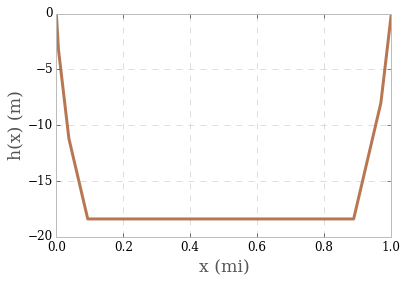In :
# plot v(x) and u(x)
fig,ax = plt.subplots()
c1 = "#e41a1c"
c2 = "#377eb8"
ax.plot( xs, tracev*mphfact, lw=2, alpha=0.8, c=c1 );
ax.set_xlabel(r"$x$ (mph)");
for tl in ax.get_yticklabels():
tl.set_color(c1)
ax.set_ylabel(r"$v(x)$ (m/s)", color=c1);
ax2 = ax.twinx()
ax2.plot( xs, traceu, lw=2, alpha=0.8, c=c2 );
for tl in ax2.get_yticklabels():
tl.set_color(c2)
ax2.set_ylabel(r"$u(x)$ (m/s$^2$)", color=c2);
ax.set_xlim((0,1609));
ax2.grid('off');
align_yaxis(ax,0,ax2,0);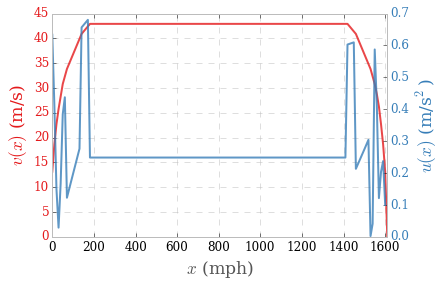In :
# plot v(t) and x(t)
fig,ax = plt.subplots()
c1 = "#e41a1c"
c3 = "#984ea3"
tts = np.r_[0,ts]
ax.plot( tts, tracev*mphfact, lw=2, alpha=0.8, c=c1 );
ax.set_xlabel(r"$t$ (s)");
for tl in ax.get_yticklabels():
tl.set_color(c1)
ax.set_ylabel(r"$v(t)$ (m)", color=c1);
ax2 = ax.twinx()
ax2.plot( tts, xs, lw=2, alpha=0.8, c=c3 );
for tl in ax2.get_yticklabels():
tl.set_color(c3)
ax2.set_ylabel(r"$x(t)$ (m/s)", color=c3);
ax.set_xlim((0,ts.max()));
ax2.grid('off');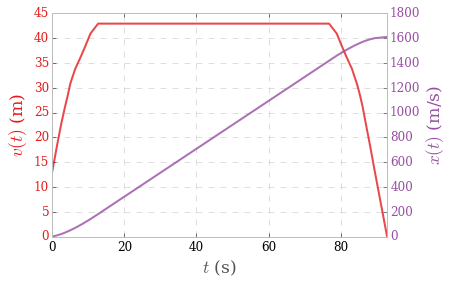In :
# plot fuel consumption
fig,ax = plt.subplots()
c4 = "#4daf4a"
consump = r(v, traceu[:-1], traceh[:-1])/v * dx * 100 * gallonperj
ax.plot( xs[:-1]/1609, consump, lw=3, alpha=0.8, c=c4 );
ax.set_ylabel("fuel use (gallons/100 mi)");
ax.set_xlabel("x (mi)");
gallons = consump.sum()/100.0
mpg = 100./consump.sum()
ax.set_title("tot={:0.2g} ga={:.1f} mpg".format(gallons, mpg));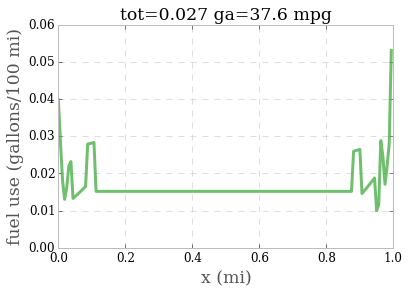In [ ]: